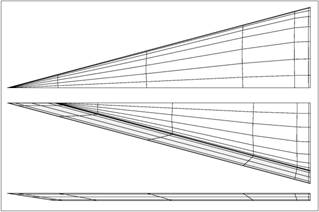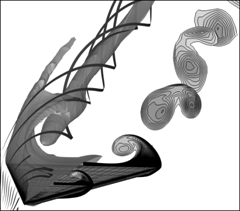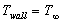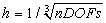Problem C2.4. Laminar Flow around a Delta Wing

Overview

A laminar flow at high angle of attack around a delta wing with a sharp leading edge and a blunt trailing edge. As the flow passes the leading edge it rolls up and creates a vortex together with a secondary vortex. The vortex system remains over a long distance behind the wing. This problem is aimed at testing high-order and adaptive methods for the computation of vortex dominated external flows. Note, that methods which show high-order on smooth solutions will show about 1st order only on this test case because of reduced smoothness properties (e.g. at the sharp edges) of the flow solution. Finally, also h-adaptive, and hp-adaptive computations can be submitted for this test case.

Governing Equations

The governing equation is the 3D Navier-Stokes equations with a constant ratio of specific heats of 1.4 and Prandtl number of 0.72. The viscosity is assumed a constant.

Flow Conditions

Subsonic viscous flow with M= 0.3, and α = 12.5°, Reynolds number (based on a mean cord length of 1) Re=4000.

Geometry

The geometry is a delta wing with a sloped and sharp leading edge and a blunt trailing edge. The geometry can be seen from Fig. 5 which shows the top, bottom and side view of the half model of the delta wing.

Half model:Figure 4: Left: Top, bottom and side view of the half model of the delta wing. The grid has been provided by NLR within the ADIGMA project. Right: Streamlines and Mach number isosurfaces of the flow solution over the left half of the wing and Mach number slices over the right half. The figures are taken from [LH10].

Reference values

Reference area: 0.133974596  (half model)

Reference moment length: 1.0

Moment line: Quarter chord

Boundary Conditions

Far field boundary: Subsonic inflow and outflow

Wing surface: no slip isothermal wall with.

Requirements

1.     Start the simulation from a uniform free stream everywhere, and monitor the L2 norm of the density residual. Track the work units needed to achieve steady state. Compute the drag and lift coefficients cd and cl.

2.     Perform grid and order refinement studies to find “converged” cd and cl values.

3.     Plot the cd and cl errors vs. work units.

4.     Study the numerical order of accuracy according to cd and cl errors vs..  (Note, that due to the locally non-smooth solution, e.g. at the sharp edges, globally high-order methods will show about 1st order only.)

5.     Submit two sets of data to the workshop contact for this case

a)     cd and cl error vs. work units

b)     cd and cl error vs6.     The following data sets can also be submitted

a.      for sequences of locally refined meshes (h-adaptive mesh refinement) and

b.     for sequences of meshes with locally varying mesh size and order of convergence (hp-adaptive mesh refinement), possibly including improved data based on a posteriori error estimation results.

Note, that here the error-vs-work-unit data sets should take account of the additional work units possibly required

-        for auxiliary problems (like e.g. adjoint problems),

-        for the evaluation of refinement indicators or mesh metrics,

-        and for the actual mesh refinement or mesh regeneration procedure.

References

[LH10] T. Leicht and R. Hartmann. Error estimation and anisotropic mesh refinement for 3d laminar aerodynamic flow simulations. J. Comput. Phys., 229(19), 7344-7360, 2010.